## 2.27.2017

### We use the law of cosines

I was always interested in a question: how the Pythagorean theorem turns into the sum of a line segments? What I speak about? Here you look.The Pythagorean theorem and the sum of a line segments
In geometry everything is very prime. The first time we draw a right triangle and we write down a Pythagorean theorem. The second time we draw two a line segments and we write down the sum of a line segments.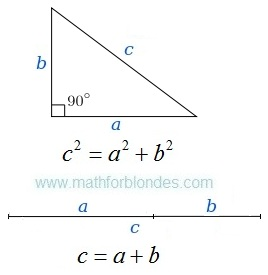Right triangle and two a line segments
How one formula turns into other formula? To see it, we use the law of cosines. We will draw the picture, we will write down conditions, we will execute transformations.Triangle and the law of cosinesRight triangle and the Pythagorean theorem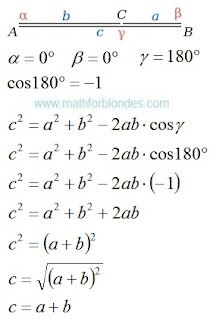The law of cosines and sum of two a line segments
We use the law of cosines and turned the Pythagorean theorem into the sum of two a line segments. Further we will consider an inverse transformation.

If you liked the publication and you want to know more, help me with working on by other publications.

## 2.25.2017

### Law of sines

The law of sines (sine law, sine formula, or sine rule) is an equation relating the lengths of the sides of a triangle (any shape) to the sines of its angles.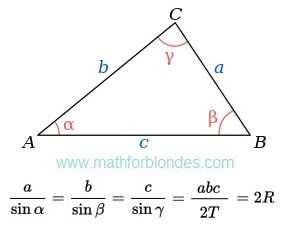The law of sines
Where:
a, b, c - are the lengths of the sides of a triangle;
α, β, γ - are the opposite angles;
T - are the area of triangle;
R - are the radius of the triangle's circumcircle.

How to use this monster? Use dress-making courses. Cut the law of sines on a part.Cut the law of sines on a part
Make a necessary formula of two parts. Use properties of proportions.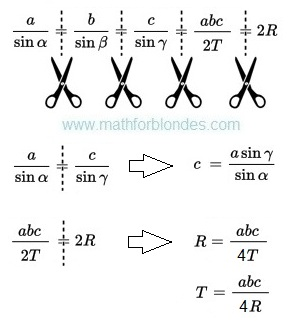Example of use of the law of sine

## 2.13.2017

### Transformations of trigonometric functions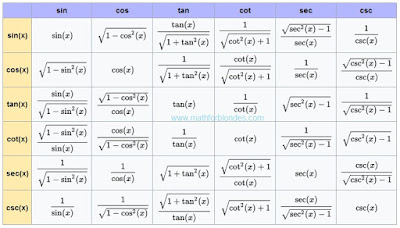Transformations of trigonometric functions
This table shows how one trigonometric functions can be transformed to other trigonometric functions. Sin, cos, tan, cot, sec, csc - all these functions can be transformed.

## 2.10.2017

### Number to the power minus one

What to do if number to the power minus one? Write down this number in a fraction denominator. Here several examples with numbers to the power minus one.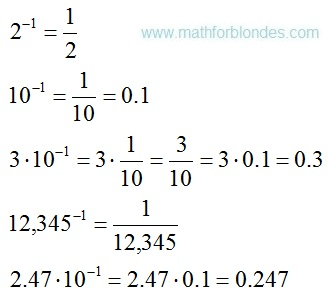Number to the power minus one
Negative exponents of numbers mean that these numbers are in a fraction denominator.Negative exponents

### Trigonometrical circle of a tangent

Last time I drew for you a unit circle of cotangent. Now you can look at a trigonometrical circle of tangent.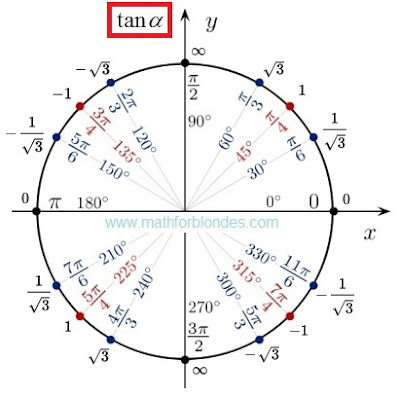Trigonometrical circle of a tangent
There is no sense here. There are angles and values of tangents. If you want to understand sense of a tangent and cotangent, look here.

## 2.08.2017

### Trigonometric tableTrigonometric table are crazy
This trigonometrical table is an example of mathematical marasmus. I am touched by exact values of a sine and cosine. No comments.

If mathematicians do not know what is trigonometric functions, let read here. If mathematicians are not able to divide into zero, let study. I like the idea of this table. I do not like its contents. I corrected this trigonometrical table.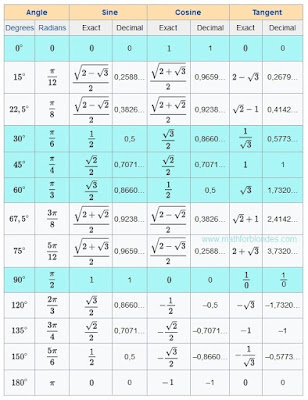Trigonometric table

The most popular angles are highlighted with blue color. 0, 30, 45, 60, 90 degrees most often occur in textbooks.Common fractions will be useful to pupils to fight against teachers. Decimal fractions will be useful physics and to engineers to calculations.

In this trigonometrical table there are no cotangents (cot, cotan, cotg, ctg, ctn). Anything terrible. There are useful formulas which will help you.Useful formulas

Sin 0, 15, 22.5, 30, 45, 60, 67.5, 75, 90, 120, 135, 150, 180 degrees in this table.
Cos 0, Pi/12, pi/8, pi/6, pi/4, pi/3, 3/8 pi, 5/12 pi, pi/2, 2/3 pi, 3/4 pi, 5/6 pi, 1 pi radians.
Tan pi/2 radians or 90 degrees it makes sense.

### Cotangent 210 degrees

Today we will consider a cotangent of 210 degrees. We will draw a unit disk and we will write values of cotangents. Here what turned out.The unit circle and cotangent
Value of a cotangent of 210 degrees same, as at a cotangent of 30 degrees. We take mathematics in hand and we check. The cotangent is the cosine divided into a sine. Values of a sine and cosine of 30 and 210 degrees can be taken here.Cotangent 210 degrees
We already know how to divide fraction into fraction. Formulas of transformation of angles of trigonometric functions.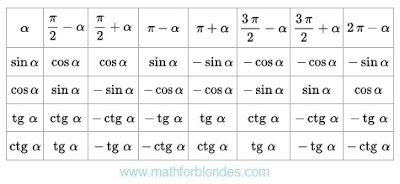Formulas of transformation of angles
These formulas will be very useful to you.

## 2.07.2017

### Signs of trigonometric functions

All trigonometric functions can have different signs. Signs of trigonometric functions depend on the coordinate system. In mathematician the Cartesian coordinate system is accepted. Four quadrants of a Cartesian coordinate system define signs of trigonometric functions.Signs of trigonometric functions
You do not speak to mathematicians, but remember. Signs are not property of trigonometric functions. Without coordinate system signs will not be. If to measure corners on another, signs will be others. In other coordinate system signs can be others.Signs of trigonometric functions are a property of the chosen coordinate system. Functions are considered in mathematician only in the Cartesian coordinate system.

## 2.06.2017

### How to divide fraction into fraction?

When performing calculations often there is a question: "How to divide fraction into fraction?". Mathematicians rules tell so:

To divide a number by a fraction, multiply that number by the reciprocal of that fraction.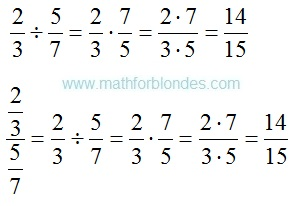How to divide fraction into fraction?
Transform fraction to division if in numerator and a denominator there are fractions. Be attentive! The numerator and denominator of fraction need to be known. The result depends on it.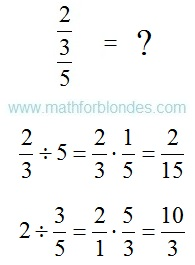The numerator and denominator

As fraction to divide into fraction. Formula of division of fractions.Formula of division of fractions
You believe that in mathematician there is a division? The fraction cannot be divided into fraction. They can only be multipled.

## 2.03.2017

### Non-inertial engine of the UFO

Last time we considered the fine-molded engine which is used in our technique. The main shortcoming - they do not save the vehicle from influence of inertial forces. Our observations show that the UFO can instantly change speed, can turn at a huge speed at right angle. It breaks the fundamental law of physics - a law of inertia. We consider that it is impossible. If such flights are impossible, means and the UFO does not exist. Our such logic.

Whether it is possible to bypass laws of inertia, without breaking them? Of course. Let's consider the principle of operation non-inertial engine of the UFO. It is the engine of absolutely other type - volume action. This engine creates internal gravitational field of the variable direction and force in the UFO. Internal gravitational field of the UFO forms selfcontained space around its design. All device UFO is as if wrapped in a cocoon.

How we overcome inertial forces? We considered our terrestrial engines earlier. In each car there are seat belts for overcoming an inertial force. Internal gravitational field of the UFO allows to overcome inertial forces at the atomic level. Each atom of a body of the extraterrestrial has the seat belt. The observer will think that inertial forces do not act on the UFO.Non-inertial engine of the UFO
It is absolutely prime further and we know it long ago. If equally effective internal gravitational field of the UFO it is equal to the gravity operating on it, then the UFO not movably. If the common force is directed up at an angle, then you need the help of trigonometry. It is a task from our, terrestrial, textbooks.

The crew of the UFO constantly is in an imponderability. The UFO constantly is in falling. For the UFO does not matter in what party and with what speed to fall. If to equip your car with the non-inertia gravitational engine, it will turn into the UFO. I do not recommend to leave limits of an Earth's atmosphere - the car housing is not calculated on it.

The UFOs non-inertial engines fall into a class of planetary engines, as well as our rocket engines. With their help it is possible to explore planets in star systems. Between star systems non-inertial engines are not suitable for travel. They have very small effectiveness on overcoming distances in space. For this purpose extraterrestrials use other type of driving - teleportation.

Why I speak about other type of driving here? The non-inertial engine and teleportation are based on one mathematical principle - the principle of closed space. In different engines different physical fields turn into closed space. The UFOs non-inertial engine closes gravitational field.

How to close space? Ask this question to our mathematicians. In reply we will hear infinitely sad song of magicians that in different sections mathematicians are different approaches to this question.

Our physicists and engineers took the first step on the way of use of physical fields for driving. In electric motors the principle of the rotating magnetic field is used. We use a magnetic levitation.

What else convinces me of reality of the UFO? Form of aircraft of extraterrestrials. You sometime heard about the cube flying in a palate? I did not hear too. Sense here not only in streamline shapes of the UFO. Physical fields have no right angles and polygonal lines.

I expect your question: if UFOs and extraterrestrials really fly over our planet, then why they do not come to us into contact? We will talk about it somehow another time.

If you liked the publication and you want to know more, help me with working on by other publications.

## 2.01.2017

### Engines and inertia

Be not surprised to the fact that now we will speak about engines and inertia. This introduction to more fascinating conversation on extra-terrestrials and their UFO. We will speak with engaging of mathematical concepts. But before to discuss extra-terrestrials, let's talk about us and the level of development of our technique.

And so, we will begin with mathematics. The matter is that all our engines are engines of dot type. Somewhere energy gathers, concentrates in one point, and then this energy is redistributed on a design of the movable device. We will not press in a jungle of mechanics and a vector algebra, we will talk about the principle.

Let's begin with the horse cart. The horse is attached to the vehicle and pulls it. The horse cart vehicle transports everything that on it is. A red arrow on the picture I noted the place of influence of strength of a horse.The horse cart
To shift the loaded horse cart, it is necessary to overcome an inertial force of the horse cart and freight. At change of speed or the direction of driving, the inertial force will force to move the horse cart in the former direction with a former speed.

Car. All saw it. All cylinders transfer the effort to a shaft in the engine of the car. This shaft rotates wheels, wheels rest against the earth and all miracle of automotive vehicles starts moving, overcoming an inertial force. Inertial forces work after collision of the car with an obstacle. Seat belts of the car counteract inertial forces.

Rocket engine. The rocket can pull the horse vehicle. The principle of the dot engine does not change. On the rocket engine we load a payload and the rocket engine pushes it to the planned purpose, overcoming inertial forces.

We use atomic energy. In the steam engine earlier we used firewood and coal. Now we use nuclear fuel. The dot principle of the steam engine remains invariable. We cannot turn gamma rays into light rays.

We learned to use fire. We know many ways as fire to get. We know many ways as fire to extinguish. But there are fires which we cannot extinguish.

Now it is possible to talk about extra-terrestrials and the UFO. Very few people doubt that for flights extra-terrestrials use the anti-gravitational engine. But how they overcome an inertial force? We transfer the principle of our terrestrial dot engines to extra-terrestrial aircraft. Can they fly by other principle? We will talk about it next time.